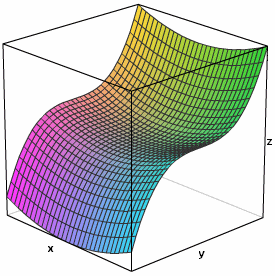Search IntMath
Close

# 3D Grapher with contour plot

By Murray Bourne, 31 Oct 2009

I recently added a 3D Grapher on IntMath.com, here: 3D and Contour Grapher.

It's Flash-based, and you can enter your own 3D function, which requires the variables x and y. The syntax for adding functions is fairly standard. You can enter examples like these:

• x^2+y^2
• e^(x-y)
• sqrt(2x^3+y^2)
• sin(x+y) [trigonometric functions require brackets]

I obtained this mathematical model for an outdoor chair using the interactive:Its function is z = f(x,y) = x2 - y3.

Have a play with the 3D and Contour Grapher and let me know what you think.

Be the first to comment below.

### Comment Preview

HTML: You can use simple tags like <b>, <a href="...">, etc.

To enter math, you can can either:

1. Use simple calculator-like input in the following format (surround your math in backticks, or qq on tablet or phone):
a^2 = sqrt(b^2 + c^2)
(See more on ASCIIMath syntax); or
2. Use simple LaTeX in the following format. Surround your math with $$ and $$.
$$\int g dx = \sqrt{\frac{a}{b}}$$
(This is standard simple LaTeX.)

NOTE: You can mix both types of math entry in your comment.